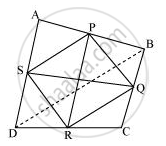# Show that the line segments joining the mid-points of the opposite sides of a quadrilateral bisect each other. - Mathematics

Show that the line segments joining the mid-points of the opposite sides of a quadrilateral bisect each other.

#### SolutionLet ABCD is a quadrilateral in which P, Q, R, and S are the mid-points of sides AB, BC, CD, and DA respectively. Join PQ, QR, RS, SP, and BD.

In ΔABD, S and P are the mid-points of AD and AB respectively. Therefore, by using mid-point theorem, it can be said that

SP || BD and SP = 1/2BD ... (1)

Similarly in ΔBCD,

QR || BD and QR = 1/2BD ... (2)

From equations (1) and (2), we obtain

SP || QR and SP = QR

In quadrilateral SPQR, one pair of opposite sides is equal and parallel to

each other. Therefore, SPQR is a parallelogram.

We know that diagonals of a parallelogram bisect each other.

Hence, PR and QS bisect each other.

Concept: The Mid-point Theorem
Is there an error in this question or solution?

#### APPEARS IN

NCERT Class 9 Maths